# Overfitting despite augmentation & label smoothing

Hi.

I’m trying to build a classifier using the Food-101 dataset. Per the recommendations of some users, I have employed label smoothing and RandTransform image augmentation techniques. However, when I try to validate my model against the test set, my accuracy is down in the dumps. Here is my notebook:

I am applying a series of RandTransforms and applying them to my data object along with Label smoothing:

``````np.random.seed(42)
batch_size = 8

path = 'images/train'
file_parse = r'/([^/]+)_\d+\.(png|jpg|jpeg)\$'
# tfms = get_transforms(do_flip=True,flip_vert=True) 80%
# tfms = get_transforms(flip_vert=False, max_lighting=0.1, max_zoom=1.05, max_warp=0.1, xtra_tfms=[cutout()])
# tfms = get_transforms(xtra_tfms=[max_zoom=1, brightness(change=(0.5-0.4, 0.5), p=0.5),contrast(scale=(1-0.6, 1), p=0.5),)
# tfms = get_transforms(do_flip=False, flip_vert=False, max_rotate:float=10.0, max_zoom:float=1.1, max_lighting:float=0.2)
# tfms = get_transforms(do_flip=False,flip_vert=False)
# tfms = get_transforms(do_flip=False, flip_vert=False, max_rotate:float=10.0, max_zoom:float=1.1, max_lighting:float=0.2, max_warp:float=0.2)

tfms = ([RandTransform(tfm=TfmCrop (crop_pad), kwargs={'row_pct': (0, 1), 'col_pct': (0, 1), 'padding_mode': 'reflection'}, p=1.0, resolved={}, do_run=True, is_random=True),
RandTransform(tfm=TfmAffine (flip_affine), kwargs={}, p=0.5, resolved={}, do_run=True, is_random=True),
RandTransform(tfm=TfmCoord (symmetric_warp), kwargs={'magnitude': (-0.2, 0.2)}, p=0.75, resolved={}, do_run=True, is_random=True),
RandTransform(tfm=TfmAffine (rotate), kwargs={'degrees': (-10.0, 10.0)}, p=0.75, resolved={}, do_run=True, is_random=True),
RandTransform(tfm=TfmAffine (zoom), kwargs={'scale': (1.0, 1.1), 'row_pct': (0, 1), 'col_pct': (0, 1)}, p=0.75, resolved={}, do_run=True, is_random=True),
RandTransform(tfm=TfmLighting (brightness), kwargs={'change': (0.4, 0.6)}, p=0.75, resolved={}, do_run=True, is_random=True),
RandTransform(tfm=TfmLighting (contrast), kwargs={'scale': (0.8, 1.25)}, p=0.75, resolved={}, do_run=True, is_random=True)],
[RandTransform(tfm=TfmCrop (crop_pad), kwargs={}, p=1.0, resolved={}, do_run=True, is_random=True)])

data = ImageList.from_folder(path).split_by_rand_pct(valid_pct=0.2).label_from_re(pat=file_parse).transform(tfms, size=224).databunch(bs = batch_size).normalize(imagenet_stats)
``````

Trying to measure for the Top-1

``````top_1 = partial(top_k_accuracy, k=1)
learn = cnn_learner(data, models.resnet50, metrics=[accuracy, top_1], loss_func = LabelSmoothingCrossEntropy(), callback_fns=ShowGraph)
``````
``````learn.lr_find()
learn.recorder.plot(suggestion=True)
``````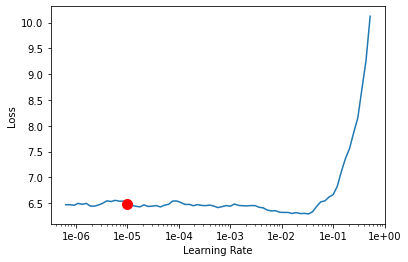My learning rate is quite high for some reason.

Running my first set of 5 epochs:

``````learn.fit_one_cycle(5, max_lr=slice(1e-05/5, 1e-05/15))
learn.save('stage-2')
``````
epoch train_loss valid_loss accuracy top_k_accuracy time
0 6.359170 5.344803 0.009967 0.009967 07:31
1 6.101181 5.079870 0.019142 0.019142 07:35
2 5.938208 4.884140 0.031551 0.031551 07:37
3 5.985401 4.818643 0.038284 0.038284 07:37
4 5.875566 4.818359 0.041056 0.041056 07:39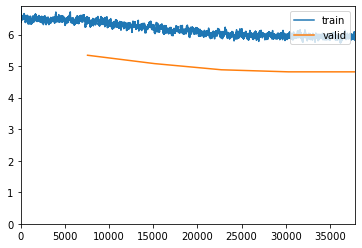Accuracy is low due to the fact that I’m label smoothing.

``````learn.lr_find()
learn.recorder.plot(suggestion=True)
``````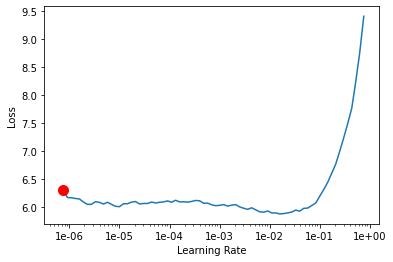``````learn.fit_one_cycle(5, max_lr=slice(1e-061/5, 1e-061/15))
learn.save('stage-3')
``````
epoch train_loss valid_loss accuracy top_k_accuracy time
0 5.968041 4.800736 0.040594 0.040594 07:36
1 5.788757 4.800635 0.040396 0.040396 07:38
2 5.955257 4.799252 0.038614 0.038614 07:38
3 5.884276 4.805166 0.041584 0.041584 07:37
4 5.940916 4.822462 0.038746 0.038746 07:38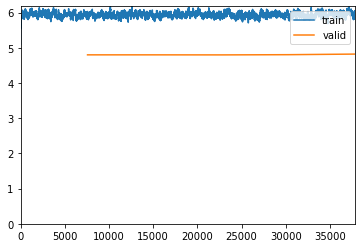Now I increased the image size from 224 to 512

``````data = ImageList.from_folder(path).split_by_rand_pct(valid_pct=0.2).label_from_re(pat=file_parse).transform(tfms, size=512).databunch(bs = batch_size).normalize(imagenet_stats)

learn = cnn_learner(data, models.resnet50, metrics=[accuracy, top_1], loss_func = LabelSmoothingCrossEntropy(), callback_fns=ShowGraph)

learn.lr_find()
learn.recorder.plot(suggestion=True)
``````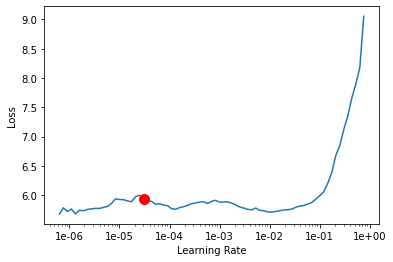``````learn.fit_one_cycle(5, max_lr=slice(1e-045/5, 1e-045/5))
learn.save('stage-4')
``````
epoch train_loss valid_loss accuracy top_k_accuracy time
0 6.075299 4.802563 0.040726 0.040726 07:36
1 5.948998 4.806314 0.040858 0.040858 07:38
2 6.004531 4.824631 0.039274 0.039274 07:39
3 5.830051 4.820912 0.038350 0.038350 07:39
4 5.971066 4.810591 0.039736 0.039736 07:37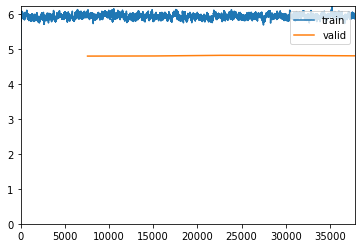Now, this is my validation step. I am now using my test set to validate my model. I am loading the saved run from stage-4. The image size remains at 512 and I am not label smoothing at this stage.

``````path = 'images'

data_test = ImageList.from_folder(path).split_by_folder(train='train', valid='test').label_from_re(file_parse).transform(size=512).databunch().normalize(imagenet_stats)

learn = cnn_learner(data, models.resnet50, metrics=[accuracy, top_1], callback_fns=ShowGraph)

learn.validate(data_test.valid_dl)
``````

And this is my lousy output:

`[4.8842754, tensor(0.0234), tensor(0.0234)]`

Few things that are bugging me:

1. I know that my image augmentation + label smoothing is faulty. I just can’t see the error I’m making.
2. When I run my epochs my accuracy fluctuates. This makes me feel like I’m either over or underfitting.
3. I don’t use `learn.freeze()` or `learn.unfreeze()`. Could that be a reason why its performing so badly?

I also tried to apply TTA and it gave me an error.

``````log_preds,y = learn.TTA(scale=1.1, ds_type=DatasetType.Valid, with_loss=False)
probs = np.mean(np.exp(log_preds),0)

accuracy(probs, y)
``````
``````---------------------------------------------------------------------------
TypeError                                 Traceback (most recent call last)
<ipython-input-17-68b28e0c5e1f> in <module>
1 log_preds,y = learn.TTA(scale=1.1, ds_type=DatasetType.Valid, with_loss=False)
----> 2 probs = np.mean(np.exp(log_preds),0)
3
4 accuracy(probs, y)

<__array_function__ internals> in mean(*args, **kwargs)

/opt/conda/envs/fastai/lib/python3.7/site-packages/numpy/core/fromnumeric.py in mean(a, axis, dtype, out, keepdims)
3330             pass
3331         else:
-> 3332             return mean(axis=axis, dtype=dtype, out=out, **kwargs)
3333
3334     return _methods._mean(a, axis=axis, dtype=dtype,

TypeError: mean() received an invalid combination of arguments - got (out=NoneType, axis=int, dtype=NoneType, ), but expected one of:
* (torch.dtype dtype)
* (tuple of names dim, bool keepdim, torch.dtype dtype)
didn't match because some of the keywords were incorrect: out, axis
* (tuple of ints dim, bool keepdim, torch.dtype dtype)
didn't match because some of the keywords were incorrect: out, axis
``````

Hi,

why do you think your model is overfitting? I think it’s just not “learning” (due to too low learning rates, also slice is imho useless if you use it on a frozen model, besides that it should be slice(lowest lr, highest lr)). Neither your training loss, nor your validation loss is decreasing.

I’d just stick to the tutorials (https://github.com/fastai/course-v3/blob/master/nbs/dl1/lesson1-pets.ipynb for example) and replace the dataset. Check the results and improve from there (labelsmoothing, different transforms etc …).

Florian

Agree with @florianl , your learning rate looks a little low. You also need to unfreeze after training the head for a few epochs. Do learn.summary() to check how many parameters of your model are being trained. Generally from what I’ve seen, label smoothing wouldn’t be responsible for such poor performance

Try train with a higher lr (3e-3?) without those additional transforms (maybe just the standard fastai transforms), unfreeze after the first 5 epochs and keep training

*In my experience i’ve found that big big pre-trained models like RexNext101or the large NLP transformer models don’t like very high lrs, but ResNet50 can be a little more accepting of higher lrs

1 Like

My LR, how it is now, gave me 81% accuracy before I attempted image augmentation

Don’t I need to freeze after my epochs to unfreeze at the next stage?

As I mentioned to Florian, my validation score was at 81% before I attempted image augmentation. I’m trying to reach >=90%.

Could you also advise me on TTA? I’m clearly doing something wrong there.

Please have a look at the notebook I posted and follow it. I am sure you will get good results with the approach in the notebook. It uses the default augmentations and there are also the right steps to train the model frozen / unfrozen and some hints to choose the right LRs.

Sure thing. Just one question. When the data object is first creator, the programmer supplied the transformations parameter with just `get_transforms()` with no arguments.

``````data = ImageDataBunch.from_name_re(path_img, fnames, pat, ds_tfms=get_transforms(), size=224, bs=bs
).normalize(imagenet_stats)
``````

Would this even work?

yes, it will use the default transforms. You can see the default parameters in the docs:

https://docs.fast.ai/vision.transform.html#get_transforms

Don’t you think I should alter the default transforms? These are images of food, at the end of the day.

the biggest advantage of fastai is, that there are VERY good defaults for most tasks especially image classification. so I’d first try the defaults and see how far you get. I don’t think that changing the transforms will give you a big (if any) improvement. But just run some experiments and compare the defaults to your altered transforms.

Sure, will definitely do that. Thank you but what do you think of my current transforms?